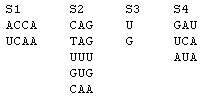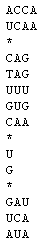CombFold - Help page[ Run | Sample Run | About ] CombFold input: Combinatorial set of RNA/DNA sequences: CombFold takes as input a description of a combinatorial set of RNA strands. There are two input formats. The first uses IUPAC code to specify a combinatorial set. For example, 5'-ARNG-3' represents the combinatorial set of 4mers in which the first and last bases are A and G, respectively, the second is a purine (A or G), and the third may be any one of A, C, G, or U, or T. For the second input format, an example of combinatorial set is given in Figure 1 below. A combinatorial set of strands is defined formally as follows. Let S1, S2, , Sk be sets of strands for some k. Within each Si, all strands should have the same length. We denote the length of the strands in set Si by ni, and the number of strands in Si by Ni. Then the combinatorial set formed from S1, S2, , Sk is the set of all strands of the form s1s2 sk where si is a strand in Si, for each i between 1 and k. Note that the length of a strand in the combinatorial set is n = n1 + n2 + ... + nk and the number of strands in the combinatorial set is N1.N2. ... Nk. Figure 1. Combinatorial set of strands.In this example, k=4 and the strands in the sets S1, S2, S3, and S4 are listed in columns. An example of a strand in the combinatorial set is UCAAGUGUUCA. The length of the strands in S1, S2, S3, and S4 are n1 = 4, n2 = 3, n3 = 1, and n4 = 3 respectively. The number of strands in S1, S2, S3, and S4 are N1 = 2, N2 = 5, N3 = 2, and N4 = 3 respectively. The length of the strands in the combinatorial set is n1+n2+n3+n4 = 11. The total number of strands in the combinatorial set is N1.N2.N3.N4 = 2.5.2.3 = 60. In the text box provided, the user must enter the strands in set S1 first, one per line. These lines should followed by a line containing only a *. Then the strands in S2 are entered, one per line, followed by a line containing a *, and so on until the strands in Sk are entered. The set of words can easily be pasted into the box from a file in the user's computer. For example, the combinatorial set corresponding to the example of Figure 1 can be entered as in Figure 2. Each strand must be a string containing the letters A, C, G, U, or T, representing the bases Adenine, Cytosine, Guanine, Uracil, and Thymine, respectively. Lower case letters (a, c, g, u, or t) are accepted. Spaces may be inserted between the characters, as well as blank lines between words, and will be removed when the calculation is performed. Figure 2. CombFold representation of the combinatorial set of Figure 1.Sequence type: The user can choose to fold the strands as RNA strands or as DNA strands. If RNA is chosen, the thermodynamic parameters of the Turner group are used (and T's are converted to U's). If DNA is chosen, the thermodynamic parameters of the SantaLucia group are used (and U's are converted to T's). Temperature: The temperature is specified in degrees Celcius and is a a real number between 0 and 100. The number must be expressed using decimal notation, e.g. 37 or 15.55. Number of strand-structure outputs: The number of strand-structure outputs can be specified as an integer between 1 and 100. Those strand-structures with the lowest free energies, up to the specified number, are output. Get results ...: The user can choose whether to view the output on a dynamically generated web page, or to receive the output via email, or both, by clicking one or both of the "as web page" and "via e-mail" boxes. If a computation takes more than 1 minute, the web interface notifies the user that the result will be sent out only via e-mail. The user must enter an email address in the box provided, in order to get the output via email. CombFold output: CombFold returns the strands in the combinatorial set with the lowest minimum free energy secondary structure at the given temperature, up to the number of combinations (i.e. strand-structures) specified by the user. For each such combination, the strand is specified by listing which strands are chosen from each set. The corresponding structure and its free energy value, enthalpy, entropy, and melting temperature are also provided. The complete strand and the corresponding minimum free energy structure are then output using dot-parenthesis notation. In this notation, a matching pair of parentheses denotes a base pair and a dot denotes an unpaired base. Links are provided to the output structures in CT (Connectivity Table) and RNAML formats. Computation time: The CPU time used to calculate the results is presented. Our current server machine is a PC with dual Intel Xeon 2GHz CPUs with 512 KB CPU cache each, and 4GB of RAM running Redhat Linux, Version 2.4.18 SMP. On an input with 10 sets, two words in each set, and all words having length 16, the computation time is less than 400 CPU seconds. On an input with 8 sets, 8 words in each set, and all words having length 4, the computation time is less than 700 CPU seconds. To keep the load on our server manageable and response times of the online services reasonably low, we limit the length of the input sequences for the online version of CombFold to the maximal processing time to 1 hour of wall-clock time (using wall-clock time in this context means that the maximal CPU time available for processing the given input may be reduced when the server load is high). If the maximal processing time is exceeded, the server notifies the user that the output has not been calculated and recommends that the user try at another time. Query ID: Identifier of the query - this is primarily used for maintainance and debugging purposes. Send these results by e-mail to ...: Allows the user to send out the results via e-mail (primarily to themselves). Specify only one e-mail address in the field and press the "Send" button to send out the e-mail version of the results webpage.